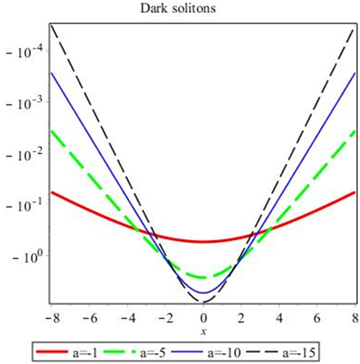Turkish Journal of Analysis and Number Theory
ISSN (Print): 2333-1100 ISSN (Online): 2333-1232
Journal Browser
Article Metrics
• 2506
Views
• 3689
Saves
• 0
Citations
• 0
Likes
Export Article
Turkish Journal of Analysis and Number Theory. 2020, 8(4), 70-74
DOI: 10.12691/tjant-8-4-1
Open AccessArticle

### New Meromorphic Solutions of the Cubic Nonlinear Schrödinger Equation by Using the Complex Method

1School of Mathematics and Information Sciences, Guangzhou University, Guangzhou, China

Pub. Date: August 22, 2020

Cite this paper:
Guoqiang Dang. New Meromorphic Solutions of the Cubic Nonlinear Schrödinger Equation by Using the Complex Method. Turkish Journal of Analysis and Number Theory. 2020; 8(4):70-74. doi: 10.12691/tjant-8-4-1

### Abstract

We show abundant simply periodic solutions, trigonometric solutions, hyperbolic function solutions and Weierstrass elliptic solutions of the reduction of the cubic nonlinear Schrödinger equation by the complex method with Painlevé analysis, and some solutions appear to be new. At last, we give some computer simulations to illustrate our main results.This work is licensed under a Creative Commons Attribution 4.0 International License. To view a copy of this license, visit http://creativecommons.org/licenses/by/4.0/

### FiguresFigure of 3

### References:

  A. M. Wazwaz, Reliable analysis for nonlinear schrödinger equations with a cubic nonlinearity and a power law nonlinearity, Mathematical and Computer Modelling, 43 (1) (2006) 178-184.  A. M. Wazwaz, Exact solutions for the fourth order nonlinear schrödinger equation with cubic and power law nonlinearity, Mathematical and Computer Modelling, 43 (7) (2006) 802-808.  A. M. Wazwaz, Partial Differential Equations and Solitary Waves Theory, Higher Education Press, Beijing, 2009.  S. M. Moawad, New exact analytic solutions for stable and unstable nonlinear schrödinger equations with a cubic nonlinearity, International Journal of Mathematical Analysis, 5 (20) (2011) 953-974.  E. M. Zayed, A. G. Al-Nowehy, Jacobi elliptic solutions, solitons and other solutions for the nonlinear schrödinger equation with fourth-order dispersion and cubic-quintic nonlinearity, European Physical Journal Plus, 132 (2017) 475.  B. Hong, D. Lu, Y. Liu, Homotopic approximate solutions for the general perturbed nonlinear schrödinger equation, Mathematical and Computational Applications, 20 (1) (2015) 50-61.  A. Liemert, A. Kienle, Fractional schrödinger equation in the presence of the linear potential, Mathematics, 4 (2) (2016) 31.  W. Yuan, Y. Li, J. Lin, Meromorphic solutions of an auxiliary ordinary differential equation using complex method, Mathematical Methods in the Applied Sciences, 36 (13) (2013) 1776-1782.  A. Eremenko, Meromorphic traveling wave solutions of the kuramoto-sivashinsky equation, Journal of Mathematical Physics Analysis Geometry, 2 (3) (2005) 278-286.  W. Yuan, Y. Wu, Q. Chen, H. Yong, All meromorphic solutions for two forms of odd order algebraic differential equations and its applications, Applied Mathematics and Computation, 37 (10) (2014) 1553-1560.  R. Conte, The Painlevé approach to nonlinear ordinary differential equations, in: The Painlevé Property, One Century Later, Springer, New York, 1999.